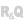WINDEVWEBDEVWINDEV MobileOthersgeoDistance (Function)
In french: geoDistance
Returns the distance between the two locations. This distance is expressed in meters by default.
Example
pos1 is géoPosition
pos1.Latitude = 1.442951
pos1.Longitude = 43.604363

pos2 is géoPosition
pos2.Latitude = 2.505874
pos2.Longitude = 50.458744

InfoBuild("The distance between the two locations is %1 meters.", géoDistance(pos1, pos2))
Syntax
<Result> = geoDistance(<Location 1> , <Location 2> [, <Unit>])
<Result>: Real
Approximate distance between the two locations. This distance is expressed in the selected unit (in meters by default).
<Location 1>: geoPosition variable
Name of the geoPosition variable corresponding to the first location to compare.
<Location 2>: geoPosition variable
Name of the geoPosition variable corresponding to the second location to compare.
<Unit>: Integer constant
Unit in which the distance must be returned:
 geoKilometer Distance in kilometers. geoMeter (default value) Distance in meters. geoMile Distance in international earth miles.
Remarks
The calculation of the distance between the two locations is based on the WGS84 ellipsoid (Worldwide Geodesic System, 1984).